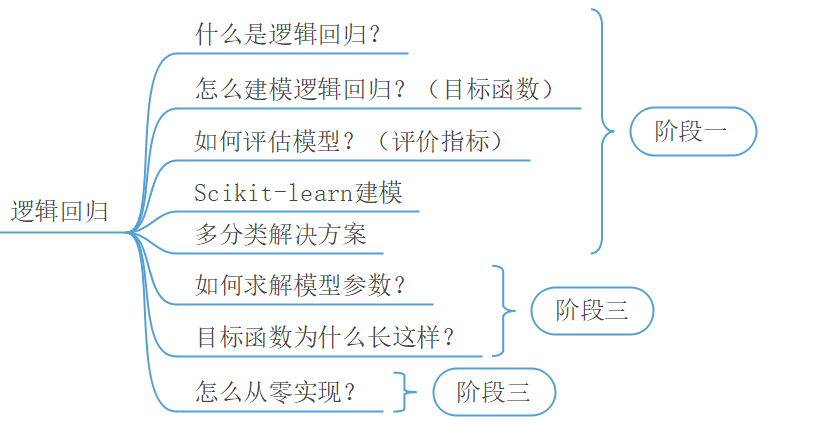### 3.1模型的建立与求解

#### 3.1.1理解逻辑回归模型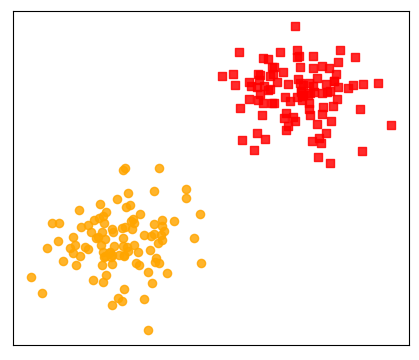#### 3.1.4逻辑回归示例代码

1) 构造数据集

xxxxxxxxxx811 from sklearn.datasets import make_blobs22 def make_data():33     centers = [[1, 1], [2, 2]]  # 指定中心44     x, y = make_blobs(n_samples=200, centers=centers,55                       cluster_std=0.2, random_state=np.random.seed(10))66     index_pos, index_neg = (y == 1), (y == 0)77     x_pos, x_neg = x[index_pos], x[index_neg]88     return x, y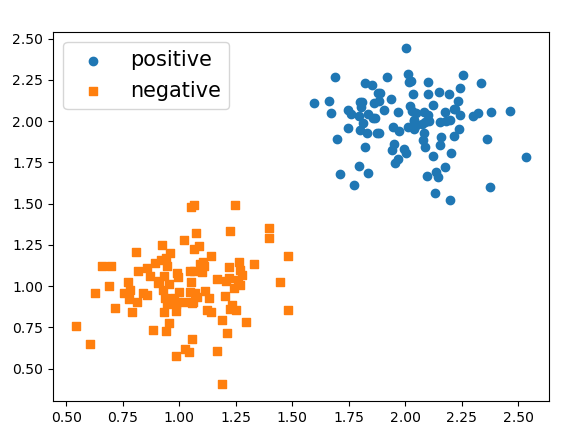2) 训练模型

xxxxxxxxxx711 from sklearn.linear_model import LogisticRegression22 def decision_boundary(x, y):33     model = LogisticRegression()44     model.fit(x, y)55     pred = model.predict([[1, 0.5], [3, 1.5]])66     print("样本点(1,0.5)所属的类标为{}\n"77             "样本点(3,1.5)所属的类标为{}".format(pred, pred))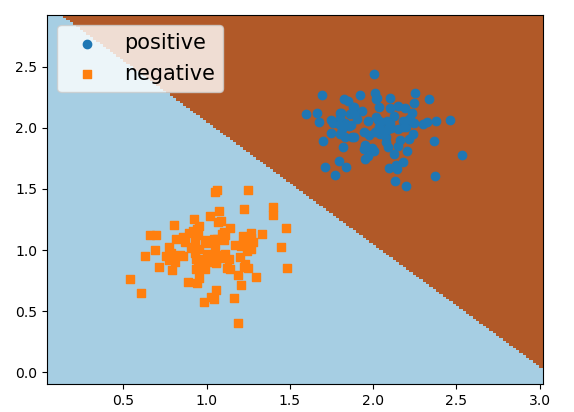### 3.2多变量与多分类

#### 3.2.2多分类逻辑回归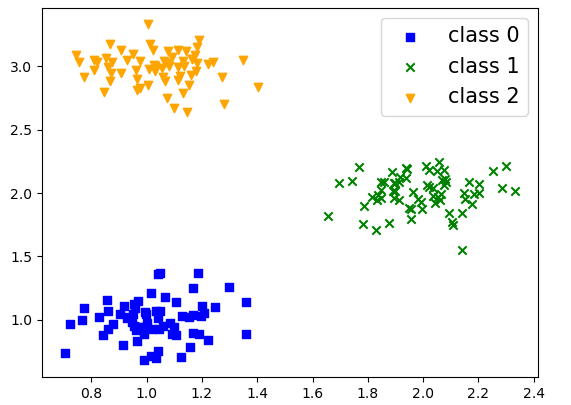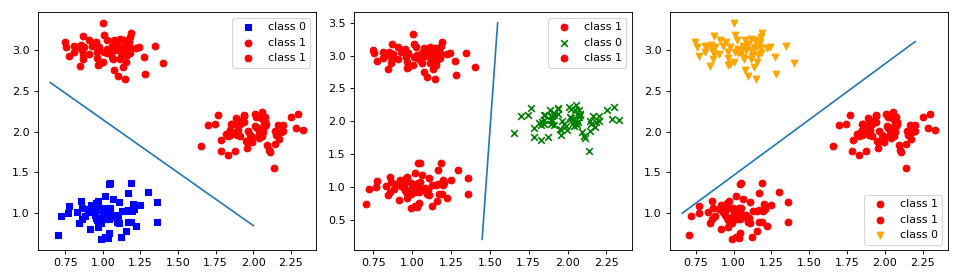#### 3.2.3多分类示例代码

1) 载入数据集

xxxxxxxxxx511 from sklearn.datasets import load_iris22 def load_data():33     data = load_iris()44     x, y = data.data, data.target55     return x, y

iris数据集一共包含有3个类别，每个类别中有50个样本，并且每个样本的有4个特征维度。同时，sklearn中也内置了很多丰富的其它数据集来方便初学者使用，具体信息可以参见官网。

2) 训练模型

xxxxxxxxxx511 def train(x, y): 22     model = LogisticRegression(multi_class='ovr')33     model.fit(x,y)44     print("得分: ", model.score(x, y))55 # 得分：0.95

### 3.3.1二分类场景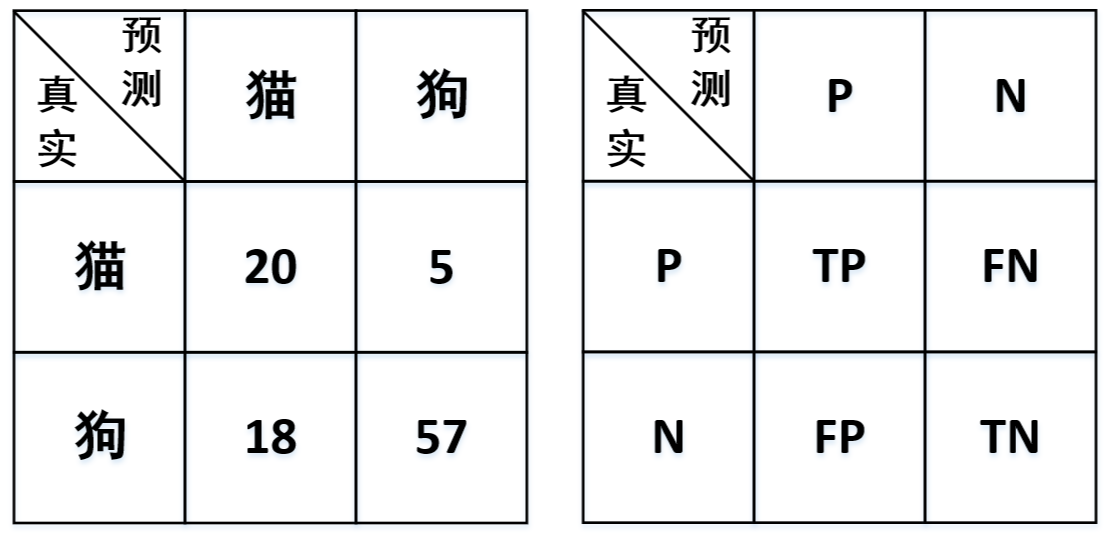• True Positive（TP）：表示将正样本预测为正样本，即预测正确；
• False Negative（FN）：表示将正样本预测为负样本，即预测错误；
• False Positive（FP）：表示将负样本预测为正样本，即预测错误；
• True Negative（TN）：表示将负样本预测为负样本，即预测正确

1) 准确率

2) $F$

• 对于类别猫来说

• 对于类别狗来说

到这里，对于4种指标各自的原理以及计算方式就算是介绍完了。但是如果要来衡量整体的精确率与召回率或者是$F$值又该怎么处理呢？对于分类结果整体的评估值，常见的做法有两种：第一种是取算术平均；第二种是加权平均

1) 算术平均

2) 加权平均

#### 3.3.2二分类指标示例代码

1) 载入数据集

xxxxxxxxxx511 from sklearn.datasets import load_breast_cancer22 def load_data():33     data = load_breast_cancer()44     x, y = data.data, data.target55     return x, y

breast_cancer数据集一共包含有2个类别（正样本与负样本），其中负样本212个，正样本357个，并且每个样本的有30个特征维度。

2) 指标计算

xxxxxxxxxx121 1 def get_acc_rec_pre_f(y_true, y_pred, beta=1.0):2 2      (tn, fp), (fn, tp) = confusion_matrix(y_true, y_pred)3 3     p1, p2 = tp / (tp + fp), tn / (tn + fn) 4 4     r1, r2 = tp / (tp + fn), tn / (tn + fp) 5 5     f_beta1 = (1 + beta ** 2) * p1 * r1 / (beta ** 2 * p1 + r1) 6 6     f_beta2 = (1 + beta ** 2) * p2 * r2 / (beta ** 2 * p2 + r2)7 7     m_p, m_r, m_f = 0.5 * (p1+p2), 0.5 * (r1+r2), 0.5 * (f_beta1 + f_beta2) 8 8     count = np.bincount(y_true)9 9     w1, w2 = count/sum(count), count/sum(count)# 计算加权平均1010     w_p, w_r,w_f=w1 * p1+w2 * p2, w1 * r1+w2 * r2, w1 *f_beta1+w2 * f_beta21111     print(f"算术平均： 精确率：{m_p},召回率：{m_r},F值：{m_f}")1212     print(f"加权平均：精确率：{w_p},召回率：{w_r},F值：{w_f}")

3) 训练模型

xxxxxxxxxx611 def train(x, y):22     model = LogisticRegression()33     model.fit(x, y)44     y_pred = model.predict(x)55     print("准确率: ", model.score(x, y))66     get_acc_rec_pre_f(y, y_pred)

xxxxxxxxxx311 准确率:  0.9522 算术平均: 精确率:0.95,召回率:0.94,F值0.9433 加权平均: 精确率:0.95,召回率:0.95,F值0.95

#### 3.3.3多分类场景

xxxxxxxxxx211 y_true = [1, 1, 1, 0, 0, 0, 2, 2, 2, 2]22 y_pred = [1, 0, 0, 0, 2, 1, 0, 0, 2, 2]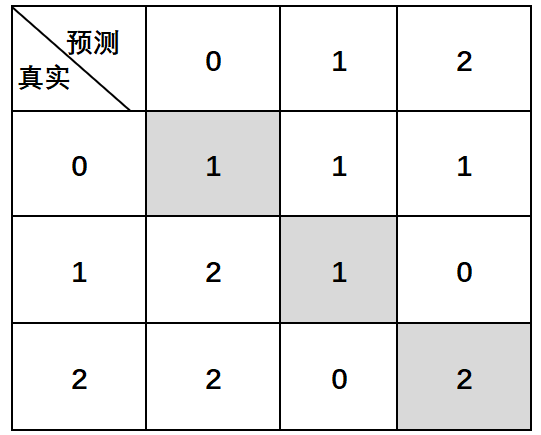1) 对于类别0来说

2) 对于类别1来说

3) 对于类别2来说

(1) 算术平均

(2) 加权平均

#### 3.3.4多分类指标示例代码

xxxxxxxxxx411 from sklearn.metrics import classification_report22 y_true = [1, 1, 1, 0, 0, 0, 2, 2, 2, 2]33 y_pred = [1, 0, 0, 0, 2, 1, 0, 0, 2, 2]44 print(classification_report(y_true, y_pred))

xxxxxxxxxx711               precision    recall  f1-score   support #分别表示精确、召回率、F值22            0       0.20      0.33      0.25         333            1       0.50      0.33      0.40         344            2       0.67      0.50      0.57         455     accuracy                           0.40        10  #模型准确率66     macro avg       0.46     0.39      0.41        10  #算术平均（宏平均）77 weighted avg       0.48      0.40      0.42        10  #加权平均

### 3.4目标函数推导

#### 3.4.1映射函数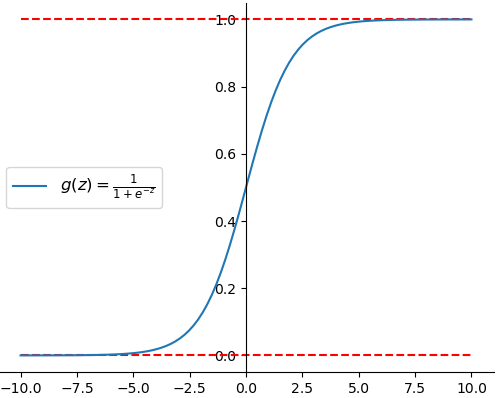Sigmoid函数的数学定义如下

xxxxxxxxxx211 def g(z):22     return 1 / (1 + np.exp(-z))

#### 3.4.4求解梯度

xxxxxxxxxx311 J(W,b)=-1/m * np.sum(y * np.log(h_x) + (1 - y) * np.log(1 - h_x)) 22 grad_w = (1 / m) * np.matmul(X.T, (h_x - y))  # [n,m] @ [m,1]33 grad_b = (1 / m) * np.sum(h_x - y)

#### 3.4.5从零实现二分类逻辑回归

1) 预测函数

xxxxxxxxxx411 def hypothesis(X, W, bias):22     z = np.matmul(X, W) + bias33     h_x = sigmoid(z)44     return h_x

xxxxxxxxxx411 def prediction(X, W, bias, thre=0.5):22     h_x = hypothesis(X, W, bias)33     y_pre = (h_x > thre) * 1#将大于阈值的True和False结果转换为1和0的标签结果44     return y_pre

2) 目标函数

xxxxxxxxxx511 def cost_function(X, y, W, bias):22     m, n = X.shape33     h_x = hypothesis(X, W, bias)44     cost = np.sum(y * np.log(h_x) + (1 - y) * np.log(1 - h_x))55     return -cost / m

3) 梯度下降

xxxxxxxxxx811 def gradient_descent(X, y, W, bias, alpha):22     m, n = X.shape33     h_x = hypothesis(X, W, bias)44     grad_w = (1 / m) * np.matmul(X.T, (h_x - y))  # [n,m] @ [m,1]55     grad_b = (1 / m) * np.sum(h_x - y)66     W = W - alpha * grad_w  # 梯度下降77     bias = bias - alpha * grad_b # 梯度下降88     return W, bias

4) 训练模型

xxxxxxxxxx911 def train(X, y, ite=200):22     m, n = X.shape  # 506,1333     W = np.random.uniform(-0.1, 0.1, n).reshape(n, 1)44     b, alpha, costs = 0.1, 0.08, []55     for i in range(ite):66         costs.append(cost_function(X, y, W, b))77         W, b = gradient_descent(X, y, W, b, alpha)88     y_pre = prediction(X, W, b)99     return costs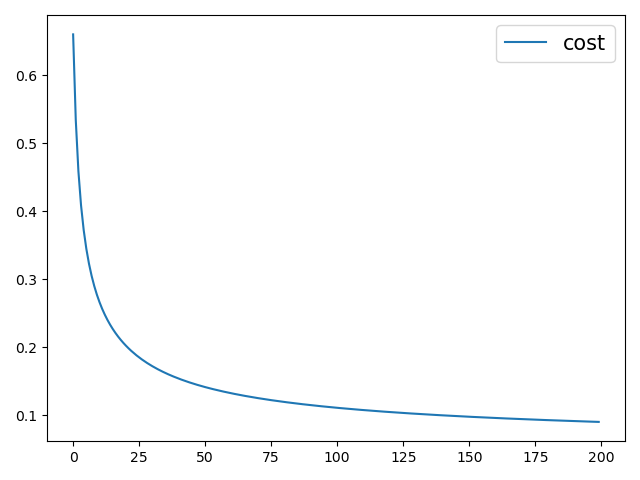#### 3.4.6从零实现多分类逻辑回归

1) 载入数据集

xxxxxxxxxx511 from sklearn.datasets import load_iris22 def load_data():33     data = load_iris()44     x, y = data.data, data.target55     return x, y

2) 定义二分类器

xxxxxxxxxx811 def train_binary(X, y, iter=200):22     m, n = X.shape  # 506,1333     W = np.random.randn(n, 1)44     b,alpha,costs = 0.3,0.5,[]55     for i in range(iter):66          costs.append(cost_function(X, y, W, b))77          W, b = gradient_descent(X, y, W, b, alpha)88     return costs, W, b

3) 训练多分类模型

xxxxxxxxxx121 1 def train(x, y, iter=1000):2 2     class_type = np.unique(y)3 3     costs, W, b = [], [], []4 4     for c in class_type:      5 5          label = (y == c) * 16 6          tmp = train_binary(x, label, iter=iter)7 7          costs.append(tmp)  8 8          W.append(tmp)      9 9          b.append(tmp)1010     y_pre = prediction(x, W, b)1111     print(classification_report(y, y_pre))1212     return costs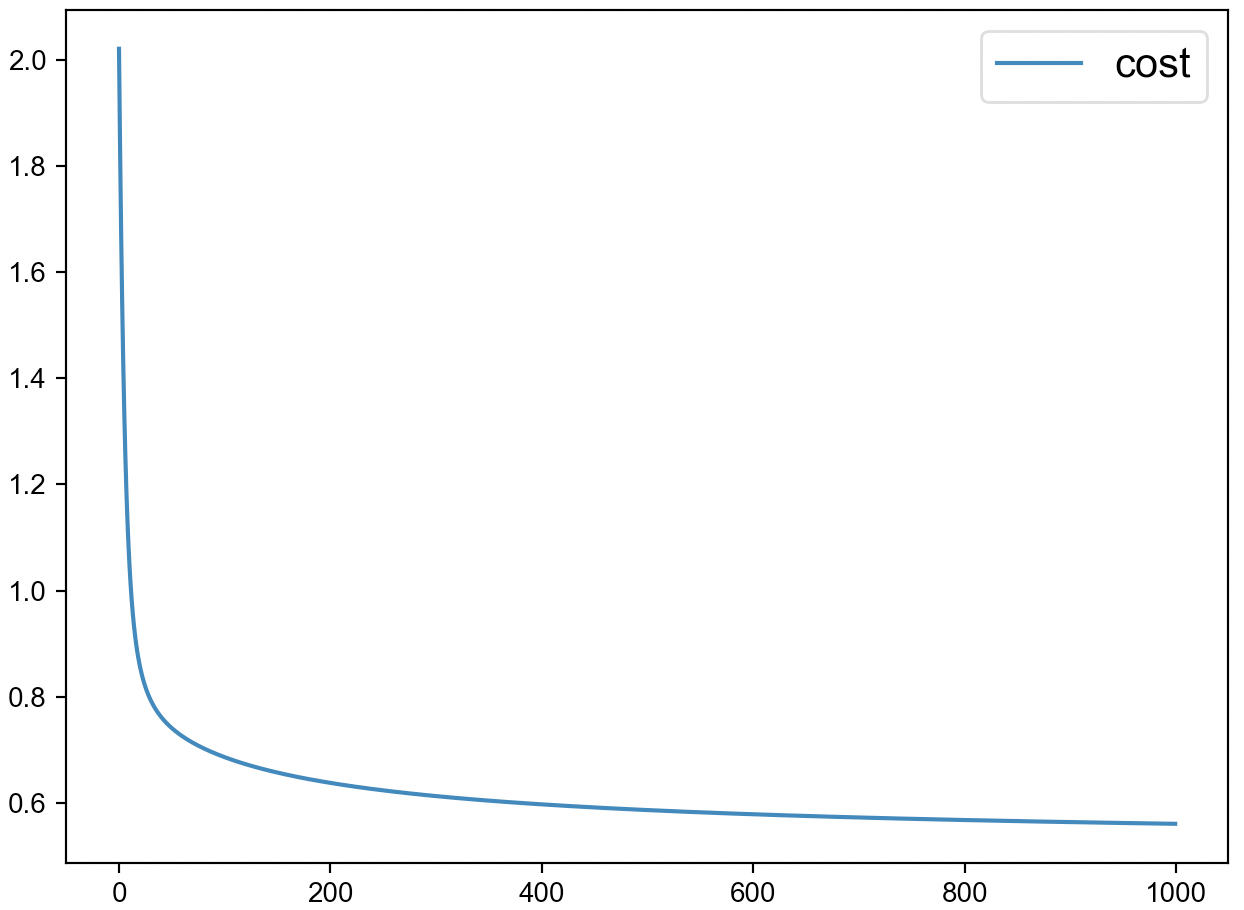#### 3.4.7小结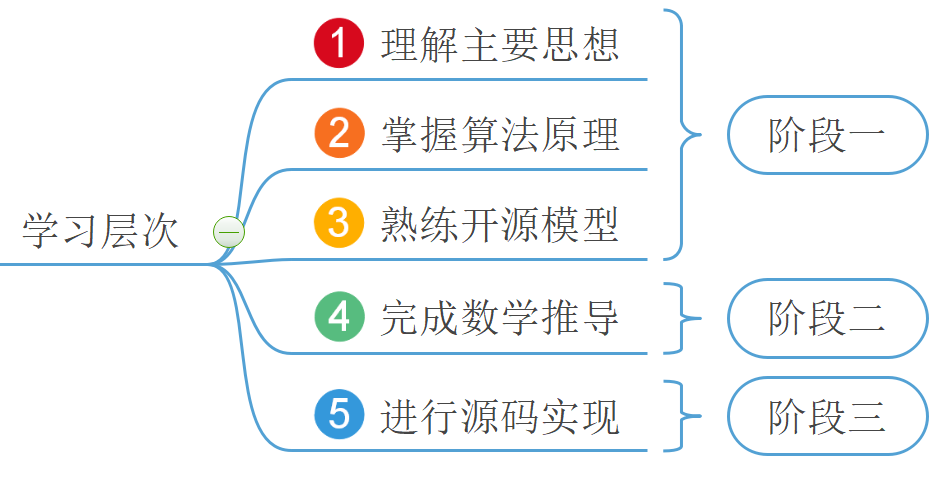### 引用

Scikit-learn: Machine Learning in Python, Pedregosa et al., JMLR 12, pp. 2825-2830, 2011.

Andrew Ng, Machine Learning, Stanford University, CS229, Spring 2019.